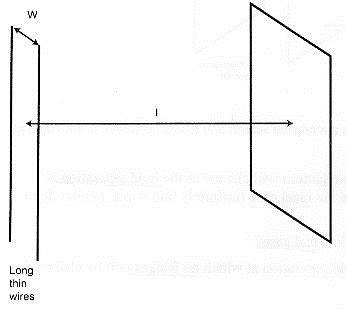# Complex Degree of Coherence (Cittert-Zernike)

• phil44

## Homework Statement

A light source consists of two long thin parallel wires, separated by a distance, W. A current is passed through the wires so that they emit light thermally. A filter is placed in front of the wires to only allow a narrow spectral range, centred at λ to propagate to a screen, a distance l from the wires.i) Obtain an expression for the complex degree of coherence using the van Cittert-Zernike theorem.

ii) Describe what would be observed on this screen.

## Homework Equations

Cittert-Zernike theorem:
Complex degree of coherence γ is given by the normalised Fourier transform of the source intensity function.

## The Attempt at a Solution

Ok so the first part is straight forward enough, treating the source intensity as a double delta function, the normalised Fourier transform of which is just a cosine function, specifically:

γ=cos $\frac{πWΔx}{λl}$ , where Δx is the separation between two points in the horizontal axis of the observation screen. Note that I'm ignoring the vertical axis at this stage.

It's the intensity seen on the screen that's confusing me. With just the double delta source directly onto a screen, I would be tempted to say the intensity distribution on the screen simply varies in a cosine fashion, as the two wire thermal sources (which are cylindrical wave sources?) interfere according to the coherence function. I feel like I might be missing something however.

Any help to clarify this would be greatly appreciated.

Last edited:
Hmm, the edit button seems to have disappeared, so sorry for the double post.

Having thought about it a bit more, what I've said might not make a whole lot of sense. The light source essentially would have random phases at each point (I think, I don't see any reason why they would be in phase across the length of the wires). So there's no real overall coherence between the two sources which can be visibly seen in the form of interference fringes. Certainly interference fringes such as those seen in diffraction wouldn't be seen I imagine. You would therefore simply see a sum of the two intensity distributions on the observation screen. I think this would physically look like two long bars in the vertical direction of maximum intensity, which diminishes outwards (gaussian?), and sums in the overlapping regions between the two wires.

Now if you were to have two pinholes as a diffraction grating, THEN the appearance of interference fringes would depend on the spacing between them, according to the coherence function mentioned in my first post. This was actually the next scenario given in the question, but I think I understand that pretty well.